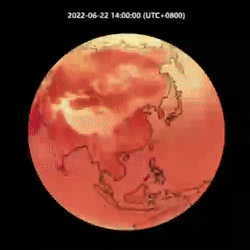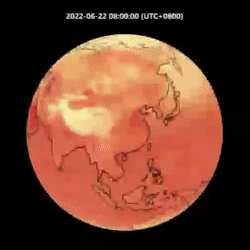# 全球气象预测开源大模型 OpenCastKit

Vachel    March 17, 2023

• 一个统一的数据处理工具，抽取ERA5数据和特征并整理成高性能训练数据格式 FFRecord
• 基于 hfai 算子hfreduce 并行通信优化的 FourCastNet 模型源码和 GraphCast 模型源码，供社区研究优化；
• 基于1979年到2022年15TB的ERA5数据，在萤火高性能集群上训练的模型参数，可以进行微调，获得高精度预测结果。

## 数据集

FourCastNet 与 GraphCast 使用了不同规模的 ERA5 数据来训练，产生的预测效果各自不同。前者仅使用了 20 个相关气象指标，包括 4 个不同位势高度下的温度、风速、相对湿度和一些近地表变量，其旨对极端天气、自然灾害进行预警；而后者使用了更加全面的数据，其包含 37 个不同位势高度下的气象指标和 5 个地表气象指标，总计 227 个指标，其旨在对气象变化进行更加全面的评估和预报。

## 模型构建和优化

### FourCastNet 数据并行

FourCastNet 模型的训练包括 pretrian、finetune 和 precipitation 三个部分。模型采用递进式，即以 $X_t$ 作为输入，预测下一步 $X_{t+1}$。一次训练输出多步，与真值对比计算 loss。如下伪代码所示：

from hfai.datasets import ERA5
from haiscale.ddp import DistributedDataParallel
from torch.utils.data.distributed import DistributedSampler

model = FourCastNet(args).cuda()
model = DistributedDataParallel(model)

data = ERA5(split='train')
sampler = DistributedSamper(data, shuffle=True)

# training ...
for step, (xt0, xt1, xt2, pt2) in enumerate(dataloader):
xt1_pred = model(xt0)            # pretrain
xt2_pred = model(xt1_pred)          # finetune
pt2_pred = model(xt2_pred, precip=True)    # preciptation

pretrain_loss = criterion(xt1_pred, xt1)
finttune_loss = criterion(xt2_pred, xt2)
precip_loss = criterion(pt2_pred, pt2)

# optim ...

# stop hfreduce
model.reducer.stop()

haiscale.ddp 默认采用 hfreduce 进行通信优化，我们还可以使用优化算子，加入一行 model = hfai.nn.to_hfai(model) 代码进行进一步加速。在萤火集群上我们使用 96 张 A100 进行数据并行加速，耗时 16~17 个小时左右基本可以完成 FourCastNet 的整体训练。

### GraphCast 数据并行

from hfai.datasets import ERA5
from haiscale.ddp import DistributedDataParallel
from haiscale.pipeline import PipeDream, make_subgroups, partition
from torch.utils.data.distributed import DistributedSampler

dist.init_process_group(...)
torch.cuda.set_device(local_rank)
rank, world_size = dist.get_rank(), dist.get_world_size()

dp_group, pp_group = make_subgroups(pp_size=pp_size)
dp_rank, dp_size = dp_group.rank(), dp_group.size()
pp_rank, pp_size = pp_group.rank(), pp_group.size()

model = GraphCast_sequentail(args)
model = partition(model, pp_group.rank(), pp_group.size(), balance=[1,1,1,1,1,1,1,1])
model = DistributedDataParallel(model.cuda(), process_group=dp_group)
model = PipeDream(model, args.chunks, process_group=pp_group)

data = ERA5(split='train')
sampler = DistributedSamper(data, num_replicas=dp_size, rank=dp_rank, shuffle=True)
earth_graph = generate_graph(args)

# training ...
for step, (xt0, xt1, xt2) in enumerate(dataloader):
loss = model.forward_backward(xt0, xt1, earth_graph, criterion=criterion, labels=(xt2,))

# optim ...

# synchronize all processes
model.module.reducer.stop()
dist.barrier()

## 预测结果FourCastNet 温度预测与风力预测GraphCast 温度预测与风力预测